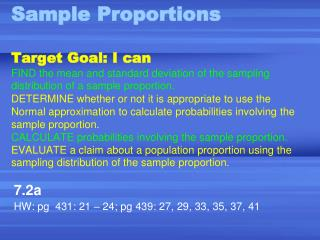Download Presentation7.2a HW: pg 431: 21 – 24; pg 439: 27, 29, 33, 35, 37, 41

# 7.2a HW: pg 431: 21 – 24; pg 439: 27, 29, 33, 35, 37, 41 - PowerPoint PPT PresentationDownload Presentation## 7.2a HW: pg 431: 21 – 24; pg 439: 27, 29, 33, 35, 37, 41

- - - - - - - - - - - - - - - - - - - - - - - - - - - E N D - - - - - - - - - - - - - - - - - - - - - - - - - - -
##### Presentation Transcript

1. Sample ProportionsTarget Goal: I canFIND the mean and standard deviation of the sampling distribution of a sample proportion.DETERMINE whether or not it is appropriate to use the Normal approximation to calculate probabilities involving the sample proportion.CALCULATE probabilities involving the sample proportion.EVALUATE a claim about a population proportion using the sampling distribution of the sample proportion. 7.2a HW: pg 431: 21 – 24; pg 439: 27, 29, 33, 35, 37, 41

2. What is the proportion of teens that know that Columbus discovered America in 1492? Gallop pollfound that 210 out of a random sample of 501 teens knew this. = 210/501 = 0.42

3. Recall: • The sample proportion is the statistic that we use to gain information about the unknown parameter p. • The parameter is the population proportion. In practice, this value is always unknown. (If we know the population proportion, then there is no need for a sample.) • The statistic is the sample proportion. • We use to estimate the value of .

4. The value of the statistic changesas the sample changes. We describe the sampling model for by • Shape • Outliers • Center • Spread

5. If our sample is an SRS of size n, then the following statements could describe the sampling model for : • The Shape is approximately normal. ASSUMPTION: Sample size is sufficiently large. CONDITION: “Rule of Thumb 2”– Use the normal approximation when

6. The center is described by the mean. Is • The spread is described by standard deviation. is or ASSUMPTION:Sample size is sufficiently large. CONDITION: “Rule of Thumb 1”– Use only when the population is at least 10 times as large as the sample. As sample size increases, the spread decreases.

7. Ex: Applying to Colleges A SRS of 1500 first year college students were asked if they applied to any other college. 35% of all first year students said they applied to other colleges. Determine the probability that the SRS of 1500 will give a result with in 2 percentage points of this true value.

8. What does this mean “with in 2 percentage points of its true value” ? We want find the probability that falls between 0.33 and 0.37. n = 1500, = 0.35 The sampling distribution has a mean = = 0.35

9. Find :“rule of thumb 1” says we must have; Test: Is thepopulation is at least 10 times as large as the sample? 10(1500) = 15,000 Yes, the population of first year students is over 1.7 million

10. is = = 0.0123

11. Is the distribution approximately normal? “Rule of Thumb 2” – Use the normal approximation when Test: = 525= 975 Yes, approximately normal.

12. Step 1:Standardize(create new statistic) P(0.33 ≤≤ 0.37)

13. Step2:Find z-scoresof= 0.33 and = 0.37 P(-1.63 ≤ z≤ 1.63)

14. Step3:Draw a picture.Use Table A or normcdf to find desired area. normcdf(-1.63,1.63) = .8968

15. State results: Almost 90% of all samples will give a result w/in 2 percentage points of the truth about the population. In Context: 90% of samples of first year college students stated they applied to other colleges will give a result within 2 % points of the true proportion.

16. Exercise:Do You Drink the Cereal Milk? A USA Today poll asked a random sample of 1012 U.S. adults what they do with the milk in the bowl after they have eaten the cereal. Of the respondents, 67% said that they drink it. Suppose that 70% of U.S. adults actually drink the milk. n = 1012 p = 0.70

17. a) Find the mean and standard deviation of the proportion of the sample that say they drink the cereal milk. μ = p = 0.70 = 0.0144 Why did we use .70 instead of .67? This time we knew the population proportion.

18. Explain why we can use the formula for the standard deviation of in this setting.(rule of thumb 1). Clearly the U.S. population is 10 times greater than 1012. • Can we use the normal approximation?(rule of thumb 2) Check: np = 1012(0.70) ≥ 10? n(1-p) = 1012(0.3) ≥ 10? Yes.

19. Find the probability of obtaining a sample of 1012 adults in which 67% or fewer say they drink the milk.Do we have any doubts about the result of this poll? 1) Find P( ≤ 0.67) Draw a picture 2) Find the z-score = 0.0188 This is a fairly unusual result if 70% of the population actually drinks the milk.

20. e) What sample size would be required to reduce the standard deviation of the sample proportion to one-half the value we found in a)? Just multiply the sample size by 4 or

21. sx If multiplying sample size by 4: 4n = 4(1012) ½ = and solve for n = 4048 adults As the sample size increases, thestandard deviation of the sampling distribution decreases. as n

22. f) If the pollsters had polled teenagers instead of adults,do you think the sample proportion greater than , equal to, or less than 0.67? Explain: It should be greater than since children tend to drink more milk than adults.